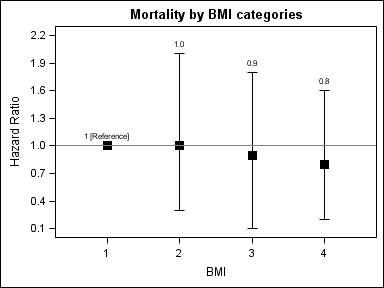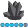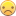## Specify location of datalabels in sgplot

Hi, I am trying to change the location of the datalabels in my plot so that they appear on top of the CIs instead of being at the center (to make nicer graphs) but I cannot find a way to do it.

I also want to modify the first datalabel so that instead of a '1' I can write '1 [Reference]' Is this option available in SAS 9.3? Thanks!

data x;

input ct ucl lcl hr;

cards ;

1     1     1     1

2     2  0.3   1

3     1.8   0.1  0.9

4     1.6  0.2  0.8

run;

proc template;

Define style styles.mystyle;

Parent=styles.journal;

Style GraphTitleText / color=black fontsize=13pt fontfamily="swiss" fontweight=bold;

Style GraphValueText / color=black fontsize=10pt fontfamily="swiss" fontweight=medium;

Style GraphLabelText / color=black fontsize=10pt fontfamily="swiss" fontweight=medium;

style GraphDataText from GraphDataText /fontsize=10pt;

end;

run;

ods html style=mystyle;

title "Mortality by BMI categories";

*title2 'Hazards Ratio and 95% CI';

proc sgplot data=x noautolegend;

scatter y=hr x=ct /

yerrorupper=ucl yerrorlower=lcl

datalabel=hr markerattrs=(size=10 symbol=squarefilled color=black)

errorbarattrs=(color=black);

refline 1  / axis=y;

yaxis minor values=(0.1 to 2.3 by 0.3) label='Hazard Ratio';

xaxis minor values=(1 to 4 by 1) label='BMI' ;

run;

1 ACCEPTED SOLUTION

Accepted SolutionsJay54
Meteorite | Level 14

## Re: Specify location of datalabels in sgplot

You can use a different column for labels.  Also you could compute the location just above the ucl, as long as you are ok setting the value in data coordinates.  If the first one is really a reference value, it need not be in the data, and you can use a reference line as suggested by Anca.data x;

length hrlabel \$15;

input ct ucl lcl hr;

hrlabel=put(hr, 3.1);

if _n_ = 1 then hrlabel="1 [Reference]";

labelloc=ucl+0.1;

cards ;

1     1     1     1

2     2  0.3   1

3     1.8   0.1  0.9

4     1.6  0.2  0.8

run;

proc print; run;

proc template;

Define style styles.mystyle;

Parent=styles.journal;

Style GraphTitleText / color=black fontsize=13pt fontfamily="swiss" fontweight=bold;

Style GraphValueText / color=black fontsize=10pt fontfamily="swiss" fontweight=medium;

Style GraphLabelText / color=black fontsize=10pt fontfamily="swiss" fontweight=medium;

style GraphDataText from GraphDataText /fontsize=10pt;

end;

run;

ods html style=mystyle;

ods listing close;

ods listing;footnote;

title "Mortality by BMI categories";

*title2 'Hazards Ratio and 95% CI';

proc sgplot data=x noautolegend;

scatter y=hr x=ct /

yerrorupper=ucl yerrorlower=lcl

markerattrs=(size=10 symbol=squarefilled color=black)

errorbarattrs=(color=black);

scatter x=ct y=labelloc / markercharacter=hrlabel;

refline 1  / axis=y;

yaxis minor values=(0.1 to 2.3 by 0.3) label='Hazard Ratio';

xaxis minor values=(1 to 4 by 1) label='BMI' ;

run;

4 REPLIES 4AncaTilea
Pyrite | Level 9

## Re: Specify location of datalabels in sgplot

Hi.

I don't know how to change the location of the data labelsHowever, to modify the first data label so that instead of a '1' to get '1 [Reference]'

this is what i suggest:

data x;

set x;

if hr = 1 & ucl = 1 & lcl = 1 then label = . ;

else label = hr;

run;

/*Then I changed the datalabel value to the 'label' variable:*/

proc sgplot data=x noautolegend tmplout = "&plot_path.\tmpl.sas";

scatter y=hr x=ct /yerrorupper=ucl yerrorlower=lcl

datalabel=label datalabelattrs = (size=10 color=black)

markerattrs=(size=10 symbol=squarefilled color=black)

errorbarattrs=(color=black);

refline 1/ label=("1 [Reference]")axis = y labelloc = inside labelpos = min;

yaxis minor values=(0.1 to 2.3 by 0.3) label='Hazard Ratio';

xaxis minor values=(1 to 4 by 1) label='BMI' ;

run;

Good luck!

Anca.DanH_sas
SAS Super FREQ

## Re: Specify location of datalabels in sgplot

You can put the labels over the UCL by making two changes:

1. In the data step, make two columns for label position and label content. The label position is derived from the UCL value.

data x;

input ct ucl lcl hr;

if (ucl = hr) then labelpos = ucl + 0.05;

else labelpos = ucl + 0.025;

label = put(hr, 3.1);

cards ;

1  1    1    1

2  2    0.3  1

3  1.8  0.1  0.9

4  1.6  0.2  0.8

run;

2. Instead of using the DATALABEL option on the scatter plot, add another scatter plot with the MARKERCHAR option to plot the label over the UCL.

proc sgplot data=x noautolegend;

scatter y=hr x=ct /

yerrorupper=ucl yerrorlower=lcl

markerattrs=(size=10 symbol=squarefilled color=black)

errorbarattrs=(color=black);

refline 1  / axis=y;

scatter y=labelpos x=ct / markerchar=label;

yaxis minor values=(0.1 to 2.3 by 0.3) label='Hazard Ratio';

xaxis minor values=(1 to 4 by 1) label='BMI' ;

run;

As for the content of the first label, you can modify the first value in the "label" column in the data step processing.

Hope this helps!

DanJay54
Meteorite | Level 14

## Re: Specify location of datalabels in sgplot

You can use a different column for labels.  Also you could compute the location just above the ucl, as long as you are ok setting the value in data coordinates.  If the first one is really a reference value, it need not be in the data, and you can use a reference line as suggested by Anca.data x;

length hrlabel \$15;

input ct ucl lcl hr;

hrlabel=put(hr, 3.1);

if _n_ = 1 then hrlabel="1 [Reference]";

labelloc=ucl+0.1;

cards ;

1     1     1     1

2     2  0.3   1

3     1.8   0.1  0.9

4     1.6  0.2  0.8

run;

proc print; run;

proc template;

Define style styles.mystyle;

Parent=styles.journal;

Style GraphTitleText / color=black fontsize=13pt fontfamily="swiss" fontweight=bold;

Style GraphValueText / color=black fontsize=10pt fontfamily="swiss" fontweight=medium;

Style GraphLabelText / color=black fontsize=10pt fontfamily="swiss" fontweight=medium;

style GraphDataText from GraphDataText /fontsize=10pt;

end;

run;

ods html style=mystyle;

ods listing close;

ods listing;footnote;

title "Mortality by BMI categories";

*title2 'Hazards Ratio and 95% CI';

proc sgplot data=x noautolegend;

scatter y=hr x=ct /

yerrorupper=ucl yerrorlower=lcl

markerattrs=(size=10 symbol=squarefilled color=black)

errorbarattrs=(color=black);

scatter x=ct y=labelloc / markercharacter=hrlabel;

refline 1  / axis=y;

yaxis minor values=(0.1 to 2.3 by 0.3) label='Hazard Ratio';

xaxis minor values=(1 to 4 by 1) label='BMI' ;

run;

## Re: Specify location of datalabels in sgplot

Thanks Anca, Dan, and Sanjay. Problem solved and nice graphs ready to present!

Discussion stats
• 4 replies
• 5995 views
• 5 likes
• 4 in conversation